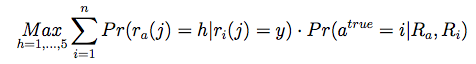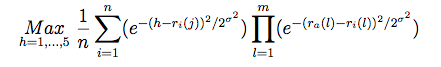# Personality Diagnosis

## Basic Algorithm

Personality diagnosis (PD) is a probability-based, model and memory hybrid algorithm. The original PD algorithm was put forth by D. Pennock et al in 2000 . Personality diagnosis works on theassumption that the active user has a hidden variable, known as a "true personality," that can accurately predict the ratings for the user on all items.

For each user in the dataset, calculate the probability that the active user is this user, given their respective rating vectors. Multiply that probability by the probability that the active user will rate the item under consideration as one of the available ratings, given what the comparison user rated the item. Sum that together over all users, and take the rating with the highest probability as the predicted rating for the active user on the item.Where h is a possible rating, n is the number of users, ra(j) is the rating of the active user on item j, and Ra is the rating vector for the active user.

This is implemented with the following equation, where the above notation holds, with the additional caveats that movies common to two users are numbered from l to m, and little sigma is a parameter.  Sigma was chosen to be 2.0 in our trials, adjusted from the experimental results found in the paper  to fit the different rating scale of our data.## Modification

The above approach uses the existing users as models for the active user, but in doing so iterates through all the known users, resulting in the complexity found in memory-based algorithms. To leverage the advantages of model-based algorithms, we can choose to iterate over only a select portion of the existing users. We incorporated the idea of a similarity table from the item-similarity algorithm, adapting it to store similar users. Using the adjusted cosine similarity measurement and similarity tables of size k=50 (see the page on item-similarity), we contrasted the results with a memory-based implementation of PD and found a significant speed-up with little loss to accuracy.

MovieLens UA test MovieLens UB test
All users RMSE = 1.11235, time = 388 seconds
RMSE = 1.12708, time = 367 seconds
50 most similar users RMSE = 1.19959, time = 27 seconds
RMSE = 1.20229, time = 28 seconds

## References

 D.Pennock, E.Horvitz, S.Lawrence, and C.L. Giles. Collaborative filtering by personality diagnosis: A hybrid memory- and model-based approach.  In Proceedings of the 16th Conference on Uncertainty in Artificial Intelligence, UAI 2000, pages 473-480, Standford, CA, 2000.